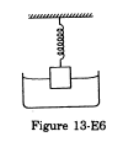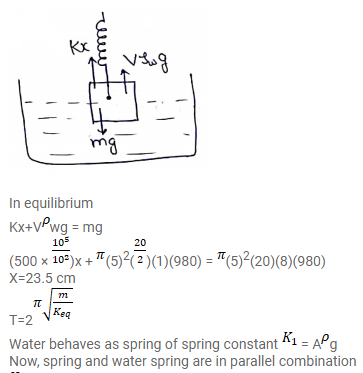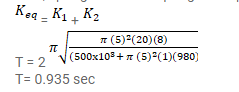# A cylindrical object of outer diameter 10 cm,

Question:

A cylindrical object of outer diameter $10 \mathrm{~cm}$, height $20 \mathrm{~cm}$ and density $8000 \mathrm{~kg} / \mathrm{m}^{3}$ is supported by a vertical spring and is half dipped in water as shown in figure(13-E6).

(a) Find the elongation of the spring in equilibrium condition.

(b) If the object is slightly depressed and released, find the time period of resulting oscillations of the object. The spring constant $=500 \mathrm{~N} / \mathrm{m}$.Solution: